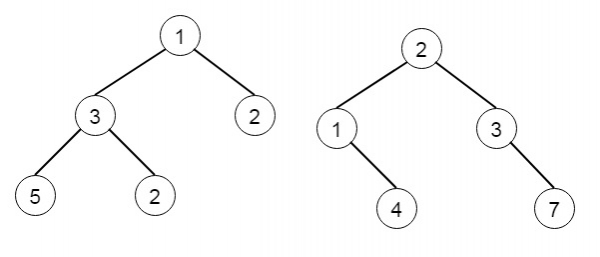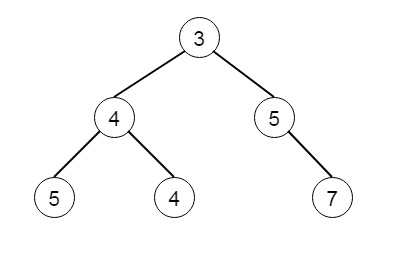# Merge Two Binary Trees in C++

Suppose we have two binary trees and consider that when we put one of them to cover the other, some nodes of the two trees are overlapped while the others are overlapping. We have to merge them into a new binary tree. The merge rule is like that if two nodes are overlapping, then sum node values up as the new value of the merged node. Otherwise, the non-empty node will be used as the node of the new tree.

So if the trees are −Then the output will be −To solve this, we will follow these steps −

• The method is mergeTrees(). This take two tree nodes n1 and n2. This is like

• if n1 is empty, and n2 is non-empty, then return n2, otherwise when n2 is empty, and n1 is non-empty, then return n1, and when both are null, return null

• value of n1 := value of n1 + value of n2

• left of n1 := mergeTrees(left of n1, left of n2)

• right of n1 := mergeTrees(right of n1, right of n2)

• return n1

## Example (C++)

Let us see the following implementation to get a better understanding −

Live Demo

#include <bits/stdc++.h>
using namespace std;
class TreeNode{
public:
int val;
TreeNode *left, *right;
TreeNode(int data){
val = data;
left = right = NULL;
}
};
void insert(TreeNode **root, int val){
queue<TreeNode*> q;
q.push(*root);
while(q.size()){
TreeNode *temp = q.front();
q.pop();
if(!temp->left){
temp->left = new TreeNode(val);
return;
}
else{
q.push(temp->left);
}
if(!temp->right){
temp->right = new TreeNode(val);
return;
}
else{
q.push(temp->right);
}
}
}
TreeNode *make_tree(vector<int> v){
TreeNode *root = new TreeNode(v);
for(int i = 1; i<v.size(); i++){
insert(&root, v[i]);
}
return root;
}
void tree_level_trav(TreeNode*root){
if (root == NULL) return;
cout << "[";
queue<TreeNode *> q;
TreeNode *curr;
q.push(root);
q.push(NULL);
while (q.size() > 1) {
curr = q.front();
q.pop();
if (curr == NULL){
q.push(NULL);
}
else {
if(curr->left)
q.push(curr->left);
if(curr->right)
q.push(curr->right);
if(curr->val == 0){
cout << "null" << ", ";
}
else{
cout << curr->val << ", ";
}
}
}
cout << "]"<<endl;
}
class Solution {
public:
TreeNode* mergeTrees(TreeNode* n1, TreeNode* n2) {
if(!n1 && n2){
return n2;
}
else if(!n2 && n1)return n1;
else if(!n1 && !n2)return NULL;
n1->val+=n2->val;
n1->left = mergeTrees(n1->left,n2->left);
n1->right = mergeTrees(n1->right,n2->right);
return n1;
}
};
main(){
Solution ob;
vector<int> v1 = {1,3,2,5};
vector<int> v2 = {2,1,3,NULL,4,NULL,7};
TreeNode *root1 = make_tree(v1);
TreeNode *root2 = make_tree(v2);
root1 = ob.mergeTrees(root1, root2);
tree_level_trav(root1);
}

## Input

[1,3,2,5]
[2,1,3,null,4,null,7]

## Output

[3, 4, 5, 5, 4, null, 7, ]